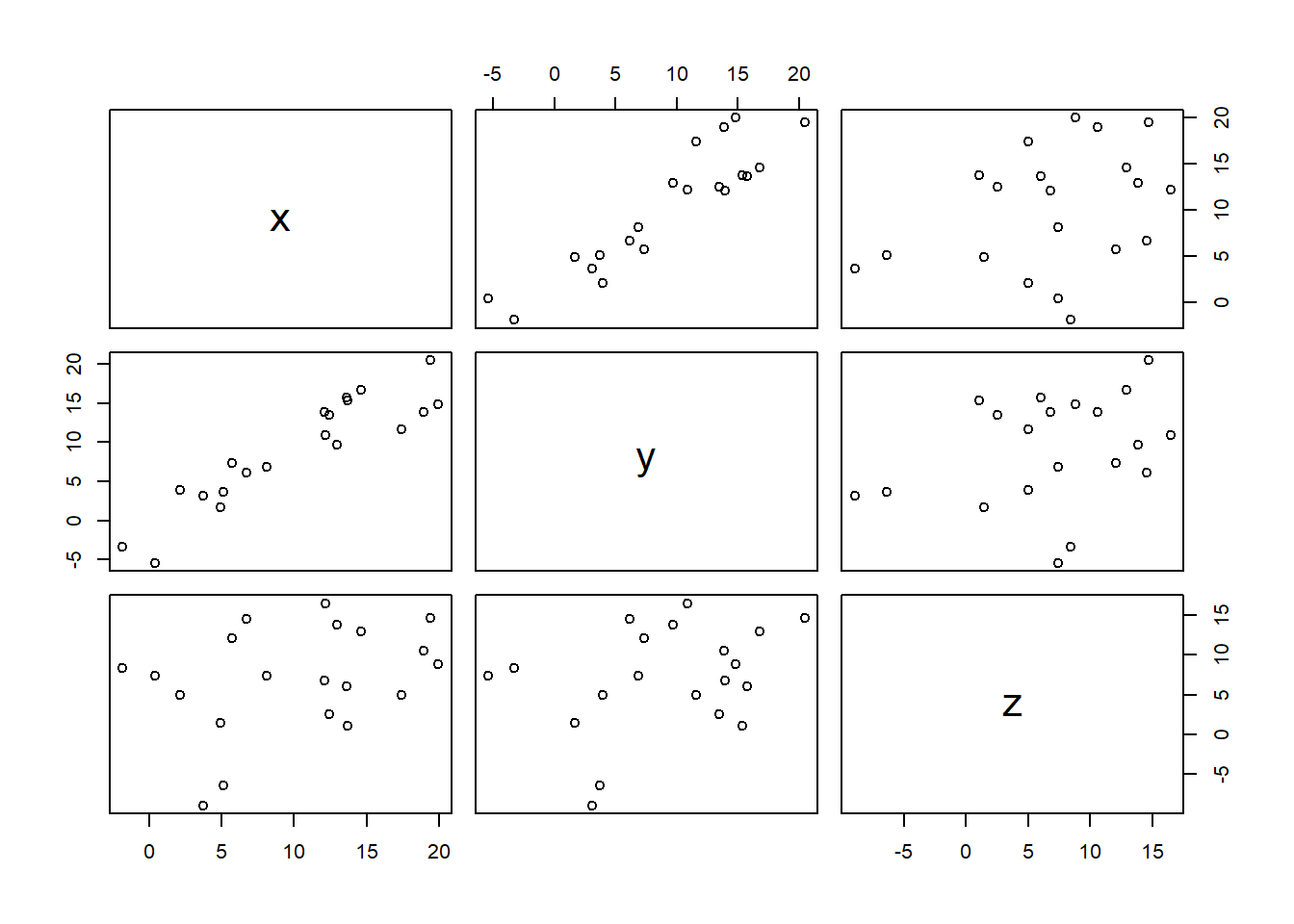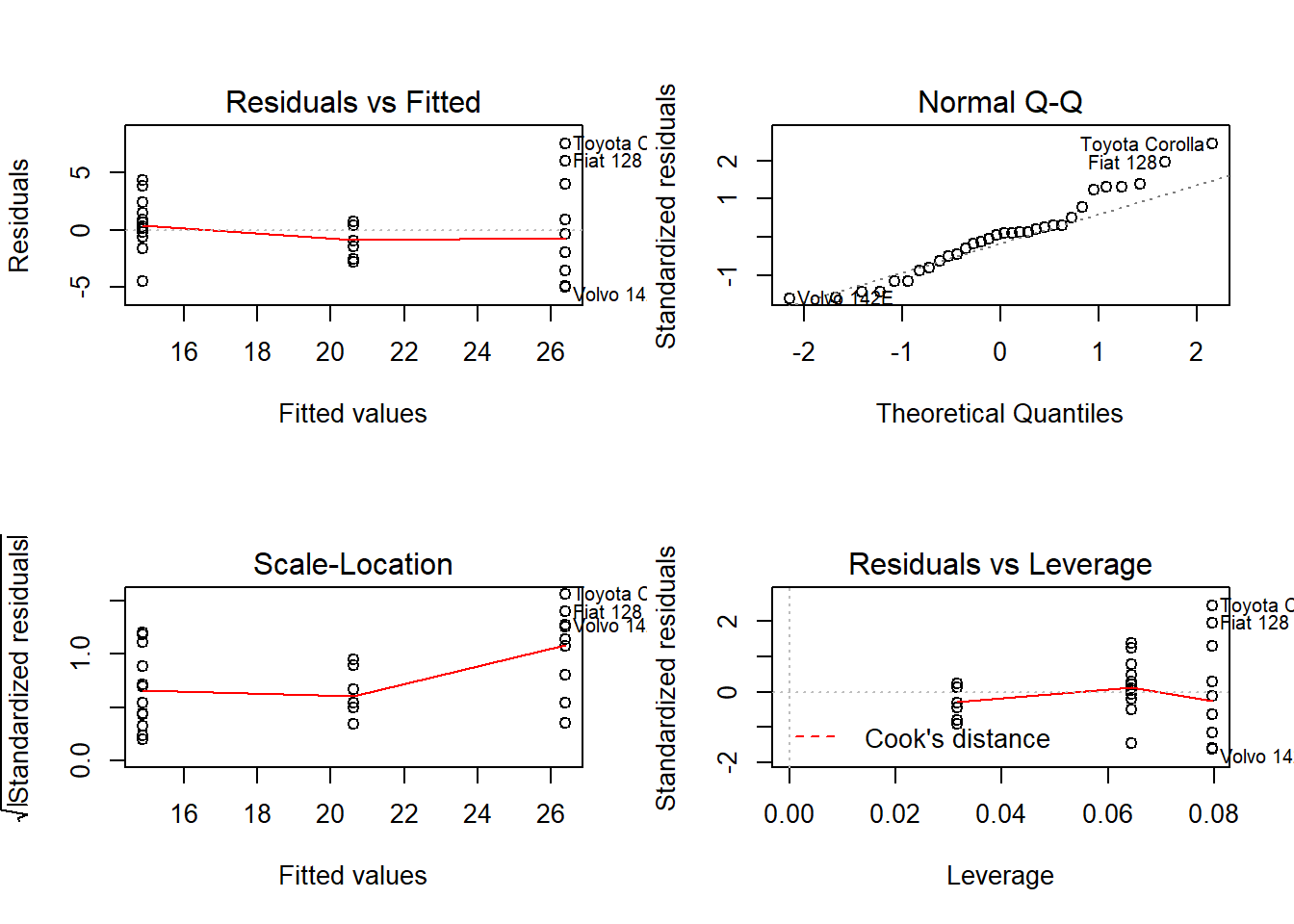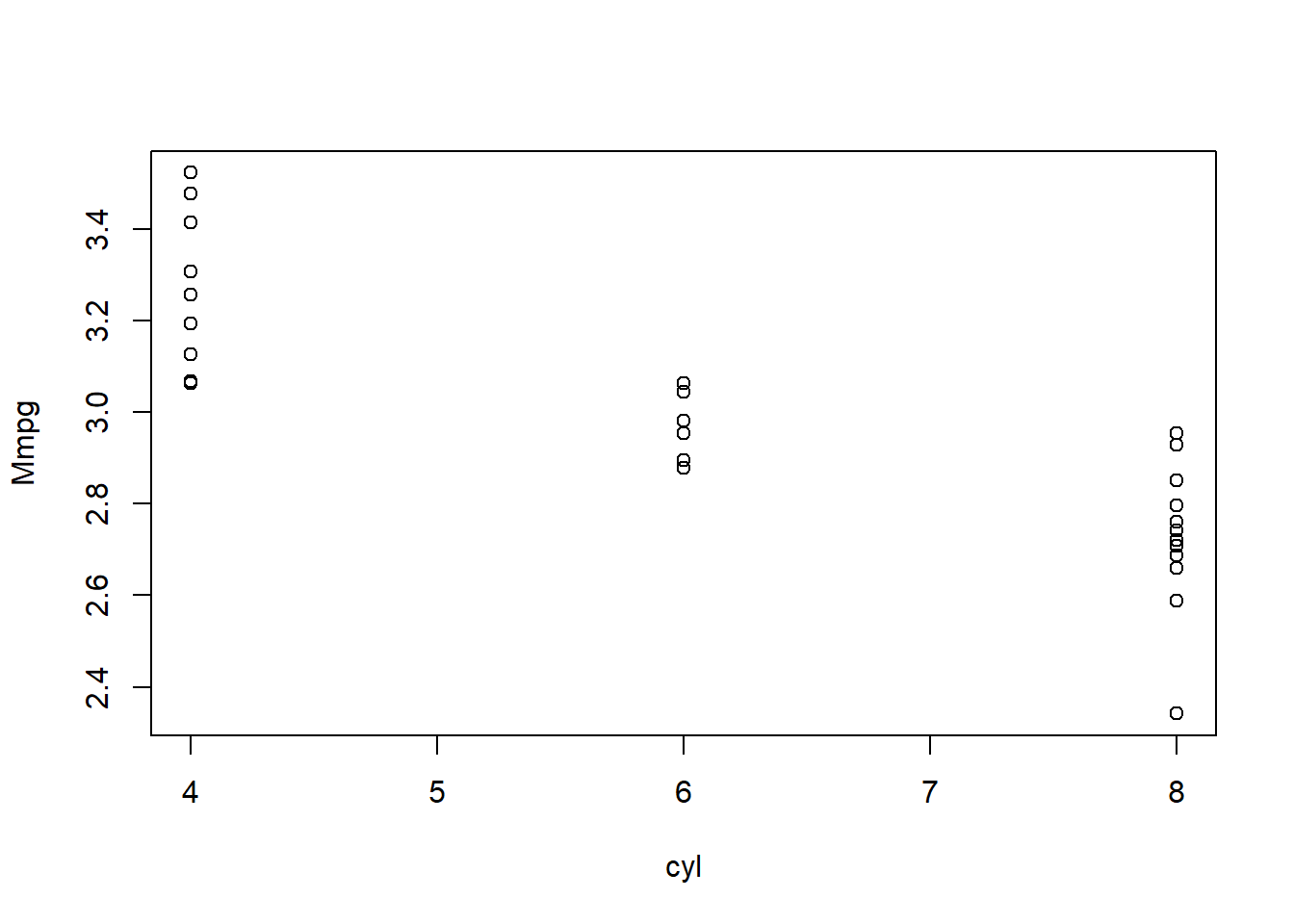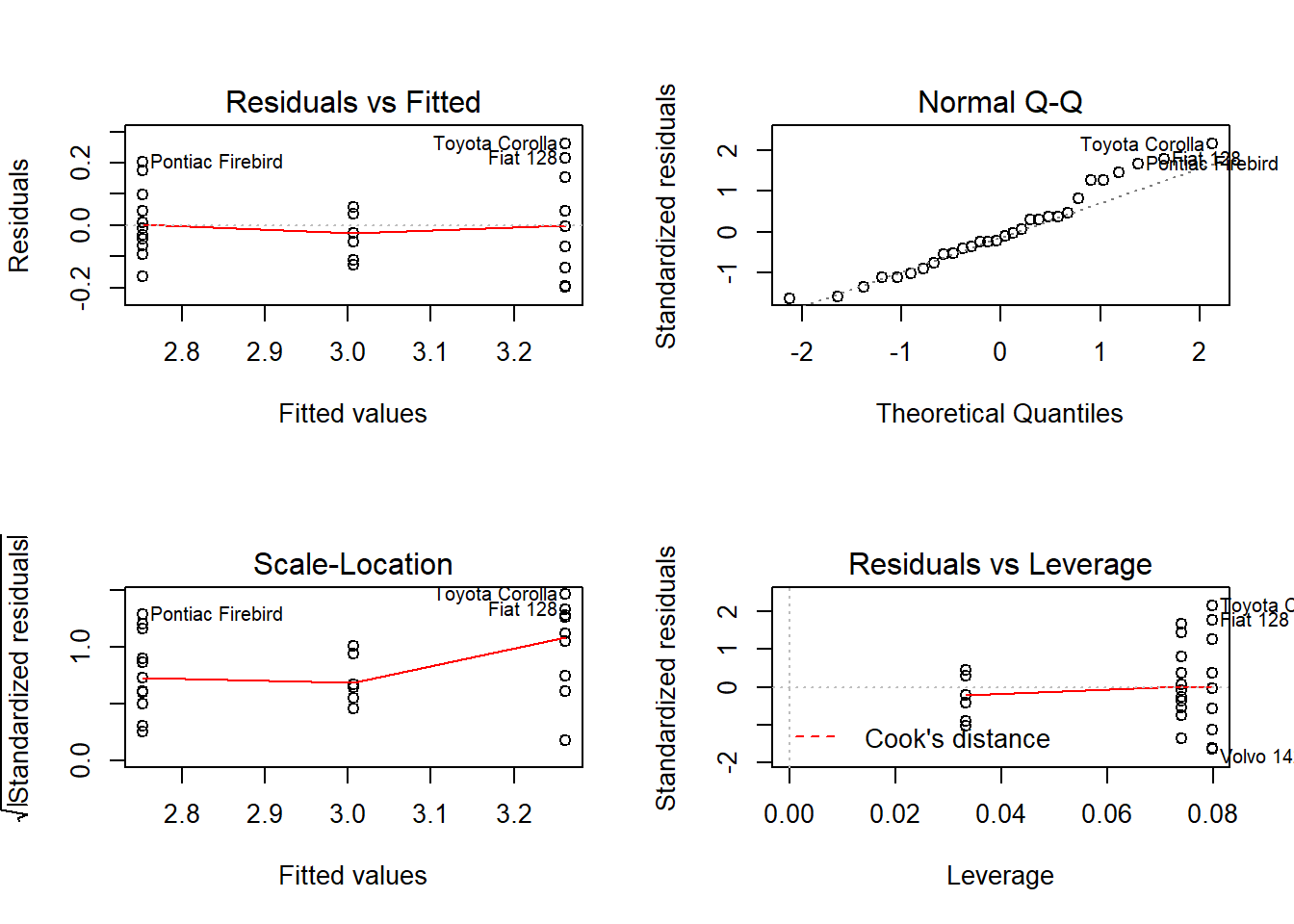## Correlation and Linear Regression

Before going into complex model building, looking at data relation is a sensible step to understand how your different variable interact together. Correlation look at trends shared between two variables, and regression look at causal relation between a predictor (independent variable) and a response (dependent) variable.

## Correlation

As mentioned above correlation look at global movement shared between two variables, for example when one variable increases and the other increases as well, then these two variables are said to be positively correlated. The other way round when a variable increase and the other decrease then these two variables are negatively correlated. In the case of no correlation no pattern will be seen between the two variable.

Let’s look at some code before introducing correlation measure:

x <- sample(1:20, 20) + rnorm(10, sd=2)
y <- x+rnorm(10,sd=3)
z <- (sample(1:20, 20)/2) + rnorm(20, sd=5)
df <- data.frame(x, y, z)
plot(df[, 1:3])From the plot we get we see that when we plot the variable y with x, the points form some kind of line, when the value of x get bigger the value of y get somehow proportionally bigger too, we can suspect a positive correlation between x and y.

The measure of this correlation is called the coefficient of correlation and can calculated in different ways, the most usual measure is the Pearson coefficient, it is the covariance of the two variable divided by the product of their variance, it is scaled between 1 (for a perfect positive correlation) to -1 (for a perfect negative correlation), 0 would be complete randomness. We can get the Pearson coefficient of correlation using the function cor():

cor(df, method = "pearson")
##           x         y         z
## x 1.0000000 0.9144510 0.3345963
## y 0.9144510 1.0000000 0.3115413
## z 0.3345963 0.3115413 1.0000000
cor(df[, 1:3],method = "spearman")
##           x         y         z
## x 1.0000000 0.8917293 0.3067669
## y 0.8917293 1.0000000 0.2766917
## z 0.3067669 0.2766917 1.0000000

From these outputs our suspicion is confirmed x and y have a high positive correlation, but as always in statistics we can test if this coefficient is significant. Using parametric assumptions (Pearson, dividing the coefficient by its standard error, giving a value that follow a t-distribution) or when data violate parametric assumptions using Spearman rank coefficient.

cor.test(df$x, df$y,method = "pearson")
##
##  Pearson's product-moment correlation
##
## data:  df$x and df$y
## t = 9.5867, df = 18, p-value = 1.703e-08
## alternative hypothesis: true correlation is not equal to 0
## 95 percent confidence interval:
##  0.7927119 0.9660476
## sample estimates:
##      cor
## 0.914451
cor.test(df$x, df$y,method = "spearman")
##
##  Spearman's rank correlation rho
##
## data:  df$x and df$y
## S = 144, p-value = 9.317e-08
## alternative hypothesis: true rho is not equal to 0
## sample estimates:
##       rho
## 0.8917293

An extension of the Pearson coefficient of correlation is when we square it we obtain the amount of variation in y explained by x (this is not true for the spearman rank based coefficient where squaring it has no statistical meanings). In our case we have around 75% of the variance in y that is explained by x.

However such results do not allow any causal explanation of the effect of x on y, indeed x could act on y in various way that are not always direct, all we can say from the correlation is that these two variables are linked somehow, to really explain and measure causal effect of x on y we need to use regression method, which will come next.

## Linear Regression

Regression is different from correlation because it try to put variables into equation and thus explain causal relationship between them, for example the most simple linear equation is written : Y=aX+b, so for every variation of unit in X, Y value change by aX. Because we are trying to explain natural processes by equations that represent only part of the whole picture we are actually building a model that’s why linear regression are also called linear modelling.

In R we can build and test the significance of linear models.

m1 <- lm(mpg ~ cyl,data = mtcars)
summary(m1)
##
## Call:
## lm(formula = mpg ~ cyl, data = mtcars)
##
## Residuals:
##     Min      1Q  Median      3Q     Max
## -4.9814 -2.1185  0.2217  1.0717  7.5186
##
## Coefficients:
##             Estimate Std. Error t value Pr(>|t|)
## (Intercept)  37.8846     2.0738   18.27  < 2e-16 ***
## cyl          -2.8758     0.3224   -8.92 6.11e-10 ***
## ---
## Signif. codes:  0 '***' 0.001 '**' 0.01 '*' 0.05 '.' 0.1 ' ' 1
##
## Residual standard error: 3.206 on 30 degrees of freedom
## Multiple R-squared:  0.7262, Adjusted R-squared:  0.7171
## F-statistic: 79.56 on 1 and 30 DF,  p-value: 6.113e-10

The basic function to build linear model (linear regression) in R is to use the lm() function, you provide to it a formula in the form of y~x and optionally a data argument.

Using the summary() function we get all information about our model: the formula called, the distribution of the residuals (the error of our models), the value of the coefficient and their significance plus an information on the overall model performance with the adjusted R-squared (0,71 in our case) that represent the amount of variation in y explained by x, so 71% of the variation in ‘mpg’ can be explain by the variable ‘cyl’.

We should first check that the models assumptions are met, indeed linear models make a few assumptions on your data, the first one is that your data are normally distributed, the second one is that the variance in y is homogeneous over all x values (sometimes called homoscedasticity) and independence which means that a y value at a certain x value should not influence other y values.

There is a marvelous built-in methods to check all this with linear models:

The par() argument is just to put all graphs in one window, the plot function is the real one. Here is the plot:

par(mfrow = c(2, 2))
plot(m1)The graphs on the first columns look at variance homogeneity among other things, normally you should see no pattern in the dots but just a random clouds of points. In this example this is clearly not the case since we see that the spreads of dots increase with higher values of cyl, our homogeneity assumptions is violated we can go back at the beginning and build new models this one cannot be interpreted… Sorry m1 you looked so great…

For the record the graph on the top right check the normality assumptions, if your data are normally distributed the point should fall (more or less) in a straight line, in this case the data are normal.

The final graph show how each y influence the model, each points is removed at a time and the new model is compared to the one with the point, if the point is very influential then it will have a high leverage value. Points with too high leverage value should be removed from the dataset to remove their outlying effect on the model.

### Transforming the data

There are a few basics mathematical transformations that can be applied to non normal or heterogeneous data, usually it is a trial and error process:

mtcars$Mmpg <- log(mtcars$mpg)
plot(Mmpg ~ cyl, mtcars)In our case this looks ok, but we can still remove the two outliers in ‘cyl’ category 8:

n <- rownames(mtcars)[mtcars$Mmpg != min(mtcars$Mmpg[mtcars\$cyl == 8])]
mtcars2 <- subset(mtcars, rownames(mtcars) %in% n)

The first line ask for row names in ‘mtcars’ (rownames(mtcars)), but only return the one where the value of the variable ‘Mmpg’ is not equal (!=) to the minimum value of the variable ‘Mmpg’ which fall in the category of 8 cylinders. Then the list ‘n’ contain all these rownames and the next step is to make a new data frame that only contain rows with rownames present in the list ‘n’.

In this stage of transforming and removing outliers from the data you should use and abuse of plots to help you through the process.

Now let’s look back at our bivariate linear regression model from this new dataset:

model <- lm(Mmpg ~ cyl, mtcars2)
summary(model)
##
## Call:
## lm(formula = Mmpg ~ cyl, data = mtcars2)
##
## Residuals:
##      Min       1Q   Median       3Q      Max
## -0.19859 -0.08576 -0.01887  0.05354  0.26143
##
## Coefficients:
##             Estimate Std. Error t value Pr(>|t|)
## (Intercept)  3.77183    0.08328  45.292  < 2e-16 ***
## cyl         -0.12746    0.01319  -9.664 2.04e-10 ***
## ---
## Signif. codes:  0 '***' 0.001 '**' 0.01 '*' 0.05 '.' 0.1 ' ' 1
##
## Residual standard error: 0.1264 on 28 degrees of freedom
## Multiple R-squared:  0.7693, Adjusted R-squared:  0.7611
## F-statistic: 93.39 on 1 and 28 DF,  p-value: 2.036e-10

Here is the plot for the model:

par(mfrow = c(2, 2))
plot(model)Again we have highly significant intercept and slope, the model explain 76% of the variance in log(mpg) and is overall significant. Now we biologist are trained to love and worship ANOVA table, in R there are several way to do it (as always an easy and straightforward way and another with more possibilities for tuning):

anova(model)
## Analysis of Variance Table
##
## Response: Mmpg
##           Df  Sum Sq Mean Sq F value    Pr(>F)
## cyl        1 1.49252 1.49252  93.393 2.036e-10 ***
## Residuals 28 0.44747 0.01598
## ---
## Signif. codes:  0 '***' 0.001 '**' 0.01 '*' 0.05 '.' 0.1 ' ' 1

The second function Anova() allow you to define which type of sum-of-square you want to calculate (here is a nice explanation of their different assumptions) and also to correct for variance heterogeneity:

Anova(model, white.adjust=TRUE)
## Analysis of Deviance Table (Type II tests)
##
## Response: Mmpg
##           Df      F    Pr(>F)
## cyl        1 69.328 4.649e-09 ***
## Residuals 28
## ---
## Signif. codes:  0 '***' 0.001 '**' 0.01 '*' 0.05 '.' 0.1 ' ' 1

You would have noticed that the p-value is a bit higher. This function is very useful for unbalanced dataset (which is our case) but need to take care when formulating the model especially when there is more than one predictor variables since the type II sum of square assume that there is no interaction between the predictors.